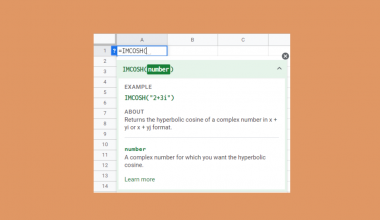# How To Use ATAN Function in Google Sheets

The ATAN function in Google Sheets is useful when you need to return the inverse tangent of a given value in radians.

The inverse tangent, also known as the arctangent, is one of the six inverse trigonometric functions. In geometry, we can use the arctangent to calculate an angle measure when the side opposite and the side adjacent to the angle are known.

The rules for using the `ATAN` function in Google Sheets are as follows:

• The function requires only a single input( the value for which to calculate the inverse tangent).
• The function then outputs the result of the arctan function in radians.

Let’s look into a real-life use-case of this function.

We’ll be using the inverse tangent function to solve a problem involving a flagpole and its shadow. Let’s say that we have a flagpole in a field. The height of the flagpole is 8 meters, and it casts a 12-meter-long shadow. What is the angle at which the sun hits the flagpole?

The flagpole and shadow form a right-angled triangle, which means we can use trigonometric functions to solve the angle. For this example, we would like to calculate the angle of theta, or α, as seen in the diagram below.With the `ATAN` function, it becomes quite easy to solve for the angle. This is because the arctangent requires knowledge of the opposite (flagpole) and adjacent (shadow) sides of an angle. Inputting the ratio of the opposite and adjacent sides into the arctan function returns the angle of theta.

Solving for angles is just one way to use this function. The `ATAN` function would be useful in engineering, astronomy, and other fields which encounter problems with unknown angles and distances.

Let’s learn how to write the `ATAN` function in Google Sheets and later test out the function with actual values.

## The Anatomy of the ATAN Function

The way we write the `ATAN` function is as follows:

`=ATAN(value)`

Let’s look at the parts of this function and understand what each term means.

• ATAN() is our `ATAN` function. It returns the inverse tangent of a value in radians.
• value refers to the value for which to calculate the inverse tangent.
• It’s important to note that the tangent is periodic, so there are many possible solutions. `ATAN` returns the solution between -π/2 and π/2.
• value must be a decimal or integer.
• The `ATAN` function returns the output in radians, not degrees.

## A Real Example of Using ATAN Function

Let’s look at an example of the `ATAN` function being used in a Google Sheets spreadsheet.

In the worksheet below, we have a table that computes the θ-angle of a right triangle, given the opposite and adjacent sides of θ. Using the `ATAN` function, we’re able to get the angle in radians. Using the `DEGREES` function, we’re able to convert it to degrees.To get the result in cell B3, we just need to use the following formula:

`=ATAN(B1/B2)`

In the second example below, we’ve also computed the case where the opposite and adjacent lengths are equal. As expected, the angle is 45 degrees, which is a property of right isosceles triangles.You can make a copy of the spreadsheet above using the link I have attached below.

If you’re ready to try out the `ATAN` function in Google Sheets, let’s begin writing it ourselves!

## How to Use ATAN Function in Google Sheets

1. First, we must select the cell where we will be writing our function. In this example, we can start with cell A4.2. Next, we’ll need to type the equal sign, followed by ‘ATAN(‘.
3. A tooltip box appears with a guide on the `ATAN` function. We can click on the arrow in the top-right-hand corner of the box to minimize it if necessary.4. The next step is typing in our argument. The inverse tangent function requires the ratio of the length of the opposite side and the adjacent side. In this case, we can simply write it as `=ATAN(B2/B3)`.
We can then simply hit the Enter key to let the function evaluate.5. We can drag the formula in cell B3 to the right to fill in the rest of the row.6. Since these results are in radians, you may also want to know the value of the angles in degrees. We can add B4, C4, and D4 as arguments into the `DEGREES` function to achieve that conversion.That’s our guide on how to use the `ATAN` function in Google Sheets. This step-by-step tutorial shows how easy it is to use the inverse tangent function to find unknown angle measurements.

You can start using the `ATAN` function in Google Sheets together with the various other Google Sheets formulas available to create great worksheet solutions for all your tasks.

Make sure to subscribe to our newsletter to be the first to know about the latest Google Sheets guides and tutorials from us.Our goal this year is to create lots of rich, bite-sized tutorials for Google Sheets users like you. If you liked this one, you'd love what we are working on! Readers receive ✨ early access ✨ to new content.

##### You May Also Like## How to Use COUNTIF Function in Google Sheets

The COUNTIF function in Google Sheets is useful if you want to count the number of times a…## How to Highlight Cells Based on Multiple Conditions in Google Sheets

A few days ago, my wife and I were organising our yearly budget for the family.  I was…## How to Use UNIQUE Function in Google Sheets

The UNIQUE function in Google Sheets is useful if you want to extract unique values from a given…## How to Use IMCOSH Function in Google Sheets

The IMCOSH function in Google Sheets is useful when you need to display the hyperbolic cosine of a…